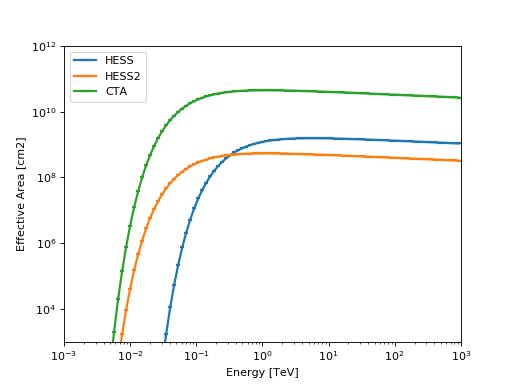# EffectiveAreaTable¶

class gammapy.irf.EffectiveAreaTable(energy_lo, energy_hi, data, meta=None)[source]

Bases: object

Effective area table.

TODO: Document

Parameters
energy_lo, energy_hiQuantity

Energy axis bin edges

dataQuantity

Effective area

Examples

Plot parametrized effective area for HESS, HESS2 and CTA.

import numpy as np
import matplotlib.pyplot as plt
import astropy.units as u
from gammapy.irf import EffectiveAreaTable

energy = np.logspace(-3, 3, 100) * u.TeV

for instrument in ['HESS', 'HESS2', 'CTA']:
aeff = EffectiveAreaTable.from_parametrization(energy, instrument)
ax = aeff.plot(label=instrument)

ax.set_yscale('log')
ax.set_xlim([1e-3, 1e3])
ax.set_ylim([1e3, 1e12])
plt.legend(loc='best')
plt.show()


(png, hires.png, pdf)Find energy where the effective area is at 10% of its maximum value

>>> import numpy as np
>>> import astropy.units as u
>>> from gammapy.irf import EffectiveAreaTable
>>> energy = np.logspace(-1, 2) * u.TeV
>>> aeff_max = aeff.max_area
>>> print(aeff_max).to('m2')
156909.413371 m2
>>> energy_threshold = aeff.find_energy(0.1 * aeff_max)
>>> print(energy_threshold)
0.185368478744 TeV


Attributes Summary

 energy max_area Maximum effective area.

Methods Summary

 evaluate_fill_nan(self, \*\*kwargs) Modified evaluate function. find_energy(self, aeff[, emin, emax]) Find energy for a given effective area. from_constant(energy, value) Create constant value effective area. from_hdulist(hdulist[, hdu]) Create from HDUList. from_parametrization(energy[, instrument]) Create parametrized effective area. from_table(table) Create from Table in ARF format. plot(self[, ax, energy, show_energy]) Plot effective area. read(filename[, hdu]) Read from file. to_hdulist(self[, name, use_sherpa]) Convert to HDUList. to_table(self) Convert to Table in ARF format. write(self, filename[, use_sherpa]) Write to file.

Attributes Documentation

energy
max_area

Maximum effective area.

Methods Documentation

evaluate_fill_nan(self, **kwargs)[source]

Modified evaluate function.

Calls gammapy.utils.nddata.NDDataArray.evaluate() and replaces possible nan values. Below the finite range the effective area is set to zero and above to value of the last valid note. This is needed since other codes, e.g. sherpa, don’t like nan values in FITS files. Make sure that the replacement happens outside of the energy range, where the EffectiveAreaTable is used.

find_energy(self, aeff, emin=None, emax=None)[source]

Find energy for a given effective area.

In case the solution is not unique, provide the emin or emax arguments to limit the solution to the given range. By default the peak energy of the effective area is chosen as emax.

Parameters
aeffQuantity

Effective area value

eminQuantity

Lower bracket value in case solution is not unique.

emaxQuantity

Upper bracket value in case solution is not unique.

Returns
energyQuantity

Energy corresponding to the given aeff.

classmethod from_constant(energy, value)[source]

Create constant value effective area.

Parameters
energyQuantity

Energy binning, analytic function is evaluated at log centers

valueQuantity

Effective area

classmethod from_hdulist(hdulist, hdu='SPECRESP')[source]

Create from HDUList.

classmethod from_parametrization(energy, instrument='HESS')[source]

Create parametrized effective area.

Parametrizations of the effective areas of different Cherenkov telescopes taken from Appendix B of Abramowski et al. (2010), see https://ui.adsabs.harvard.edu/abs/2010MNRAS.402.1342A .

$A_{eff}(E) = g_1 \left(\frac{E}{\mathrm{MeV}}\right)^{-g_2}\exp{\left(-\frac{g_3}{E}\right)}$
Parameters
energyQuantity

Energy binning, analytic function is evaluated at log centers

instrument{‘HESS’, ‘HESS2’, ‘CTA’}

Instrument name

classmethod from_table(table)[source]

Create from Table in ARF format.

Data format specification: ARF

plot(self, ax=None, energy=None, show_energy=None, **kwargs)[source]

Plot effective area.

Parameters
axAxes, optional

Axis

energyQuantity

Energy nodes

show_energyQuantity, optional

Show energy, e.g. threshold, as vertical line

Returns
axAxes

Axis

classmethod read(filename, hdu='SPECRESP')[source]

to_hdulist(self, name=None, use_sherpa=False)[source]

Convert to HDUList.

to_table(self)[source]

Convert to Table in ARF format.

Data format specification: ARF

write(self, filename, use_sherpa=False, **kwargs)[source]

Write to file.